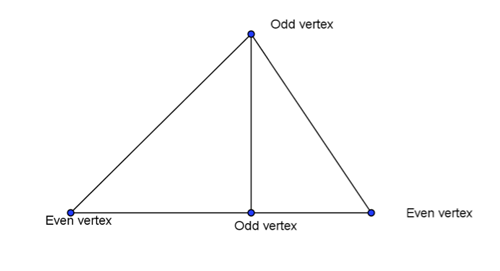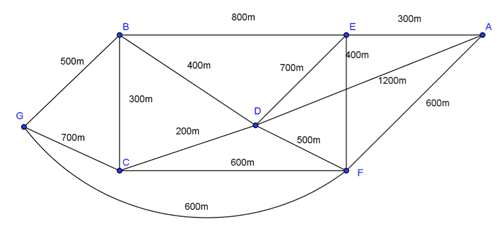Te Kete Ipurangi
Communities
Schools

### Te Kete Ipurangi user options:

Achievement objectives

What has changed:

# How to establish traversability

Which of these diagrams can be drawn without taking your pencil off the paper and without going over the same line twice?

(Note: This is the famous Konigsberg bridges question which Euler posed in 1735, and is the very first problem solved about networks.)

## Key terms relating to traversability

We can model the problems above as graphs by introducing a vertex (where two lines meet) and by regarding the lines as edges.Diagram 2

• If we can find a walk, essentially a sequence of edges, that goes over all the edges without repeating an edge, then the graph is traversable.
• Whether or not a graph is traversable depends on the number of odd vertices it has.
• The number of edges that meet at a vertex is called the degree of the vertex.
• An odd vertex is a vertex with an odd degree. An even vertex is a vertex with an even degree.Diagram 3

A graph will have an even number of odd vertices, because the sum of the degrees of all the vertices is twice the number of the edges.

A connected graph with even vertices only is traversable, and we can start and finish at the same vertex. It is called Eulerian. Further, we can choose any vertex to be the start/finish.

A connected graph with exactly two odd vertices is traversable as long as we start at one of the odd vertices and finish at the other. It is called semi-Eulerian.

A connected graph with more than two odd vertices is not traversable.

## An example of traversability: Route inspection

### Problem 1

Hone is a postie who has to cycle along all the roads shown in this network. The lengths of the roads in metres are shown on this network. Can he do this without cycling along any road twice?

All the vertices are even, so Hone is able to transverse the network. He can start and return to any point. One possible route is AEBCDFEDBGCFA. The length of this postal route is 6000 m or 6 km (the sum of the lengths of the edges).

### Problem 2

New Zealand Post add one more road to Hone’s route that goes directly between A and D. This road passes through a tunnel under the road EF so there is no vertex where these edges (AD and EF) cross on the graph.

(There are two odd vertices, A and D, so Hone could traverse the network by starting at A and finishing at D or vice versa. The distance travelled is now 7200 m.)

b) Hone would ideally like to start and finish at the same place so that he can drive to the starting point, cycle round the route, and get back to his car. Find a way Hone can do this that minimises the distance he cycles.

If he wants to do this, and start at A, he will have to traverse the graph from A to D and then find the shortest route back to A. We can see from the graph that the shortest way back from D to A is DEA, which adds 1000m to his route.

One possible way of doing this is AEBGCFEDBCDFADEA, and its length is 8200 m. There are many possible routes.

### Problem 3

New Zealand Post adds another road to Hone’s round that goes directly from G to F.Diagram 6

Hone is unable to transverse the network as there are four odd vertices, A, D, F, and G.

b) Explain how Hone could complete his round, starting and finishing at the same point.

To complete his round, and start and finish at the same point, Hone will have to repeat the edges that connect the four odd vertices: A, D, F, and G.

He can do this by repeating one of the following pairs of paths:

• G–F and A–D: The shortest route to do this is 1600m (GF and DEA)
• G–D and A–F: The shortest route to do this is 1500m (GBD and AF)
• G–A and D–F: The shortest route to do this is 1700m (GFA and DF)

The middle pairing gives the shortest extra distance.

One possible shortest route is AEBGCFEDBCDFGBDAFA, and the length is now 9300 m.

Last updated May 1, 2012